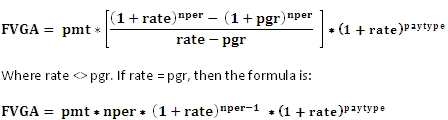# SQL Server future value of a growing annuity

FVGA

Updated: 07 February 2011

Use FVGA to calculate the future value of a growing annuity. The formula for the calculation of the future value of a growing annuity is:Syntax
SELECT [wctFinancial].[wct].[FVGA](
<@Pmt, float,>
,<@Pgr, float,>
,<@Nper, float,>
,<@Rate, float,>
,<@Pay_type, int,>)
Arguments
@Pmt
the amount of the annuity payment in the first period. @Pmt is an expression of type float or of a type that can be implicitly converted to float.
@Pgr
the periodic growth rate of the annuity. This is the percentage amount, expressed as a decimal, by which the annuity will increase in each period. @Pgr is an expression of type float or of a type that can be implicitly converted to float.
@Nper
the number of annuity payments. @Nper is an expression of type float or of a type that can be implicitly converted to float.
@Rate
the percentage rate of return, expressed as a decimal, that you expect the annuity to earn over the number of periods. The annuity payments are compounded using this value. @Rate is an expression of type float or of a type that can be implicitly converted to float.
@Pay_type
the number 0 or 1 and indicates when payments are due. @Pay_type is an expression of type int or of a type that can be implicitly converted to int. If @Pay_type is NULL it is assumed to be 0.

 Set @Pay_type equal to If payments are due 0 At the end of a period 1 At the beginning of a period

Return Type
float
Remarks
·         The FVGA value will have the same sign as @Pmt.
·         If the @Pay_type is not equal to zero, it is assumed to be 1.
·         If @Rate is equal to -1 (-100%), FVGA will fall over with a divide by zero.
·         To calculate the present value of a growing annuity, use the PVGA function.
Examples
Let’s say you make \$40,000 per year, and you plan on saving 8% of your salary in a tax-deferred account. For purposes of this example, you will make the first payment of \$3200 today and then make annual contributions. You expect your salary to increase 3% per year, and you expect your tax-deferred account to grow at 7% per year. How much will have when you retire in 40 years?
SELECT
wct.FVGA(3200     --@Pmt
,.03        --@Pgr
,40         --@Nper
,.07        --@Rate
,1          --@Pay_type
) as FVGA
This produces the following result.
FVGA
----------------------
1002583.156041

(1 row(s) affected)

One way to interpret this result is that is that in this investement scenario, you will retire as a millionaire. But, that’s in 40 years time. We can calculate what that future value is worth today by using the present value (PV) function and the present value of a growing annuity function (PVGA).
SELECT
wct.PV(0.07,40,0,-1002583.156041,0) as PV
,wct.PVGA(3200,.03,40,.07,1) as PVGA
This produces the following result.
PV                   PVGA
---------------------- ----------------------
66952.8851599541       66952.8851599539

(1 row(s) affected)

You could achieve the same result, though the SQL is more complicated, by creating the actual cash flows and then using the aggregate NFV function to calculate the net future value. Since we used a pay_type of 1, the NFV result needs to be multiplied by 1 plus the rate.
SELECT wct.NFV(.07,cf,per) * 1.07 as FVGA
FROM (
SELECT seriesvalue as per
,POWER(cast(1.03 as float), seriesValue) * 3200 as cf
FROM wct.SeriesInt(0,NULL, 1, 40, NULL)
) n
This produces the following result.
FVGA
----------------------
1002583.156041

(1 row(s) affected)

### SupportCopyright 2008-2022 Westclintech LLC         Privacy Policy        Terms of Service